#Function Repository Resource:

# FindProperColorings

Find all proper k-colorings of a specified graph

Contributed by: Daniel McDonald
 ResourceFunction["FindProperColorings"][g,k] finds all proper k-colorings of the graph g.

## Details and Options

A proper k-coloring of a graph g is a function assigning each vertex of g one of k colors such that adjacent vertices receive distinct colors.
A list of all proper k-colorings of g is returned, where each coloring is a list whose ith element is an integer from 1,,k representing the color assigned to the ith vertex in VertexList[g].

## Examples

### Basic Examples

Define a graph:

 In:=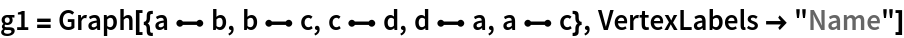Out=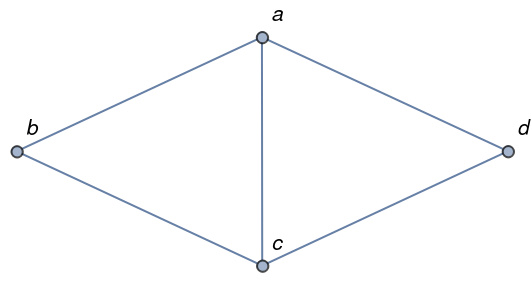Find all its proper 3-colorings:

 In:=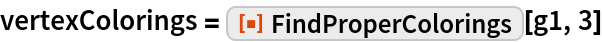Out=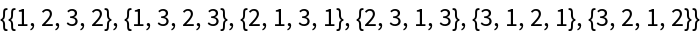Display the proper 3-colorings:

 In:=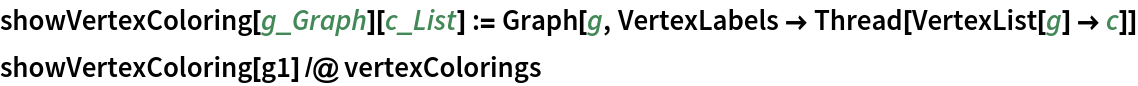Out=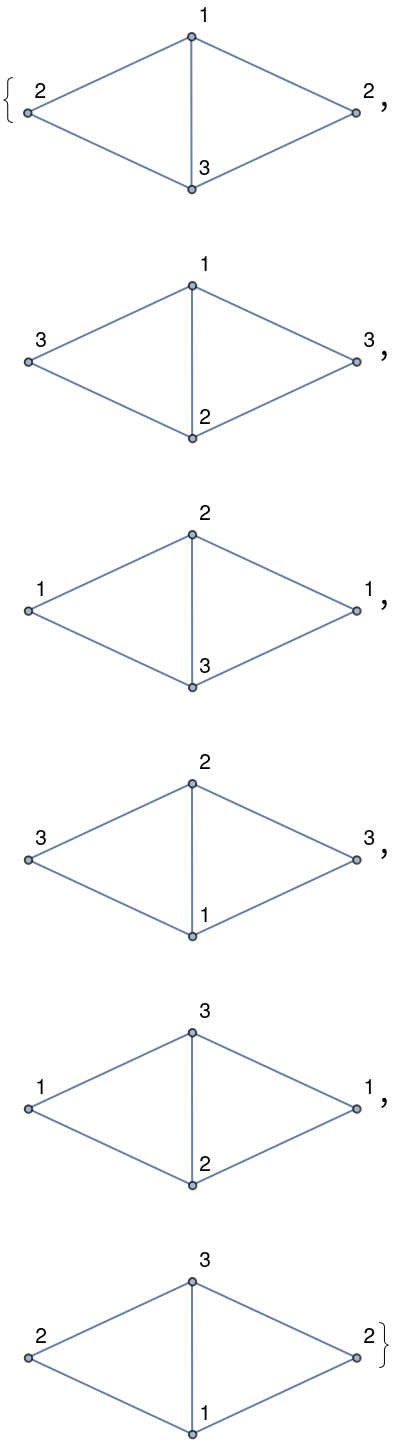### Scope

FindProperColorings works on disconnected graphs:

 In:=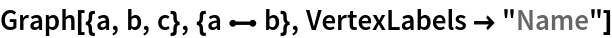Out=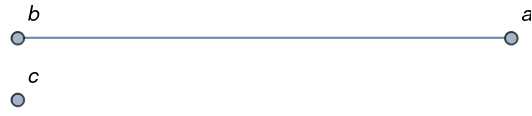In:=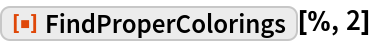Out=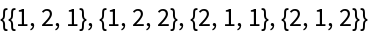In:=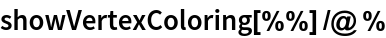Out=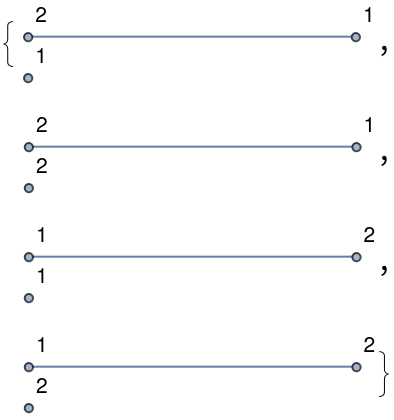FindProperColorings works on directed graphs:

 In:=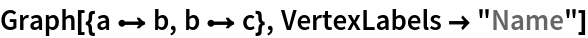Out=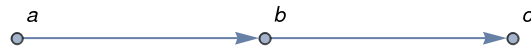In:=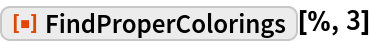Out=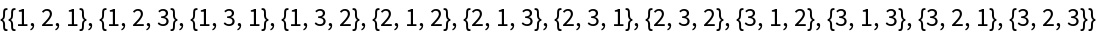In:=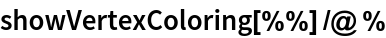Out=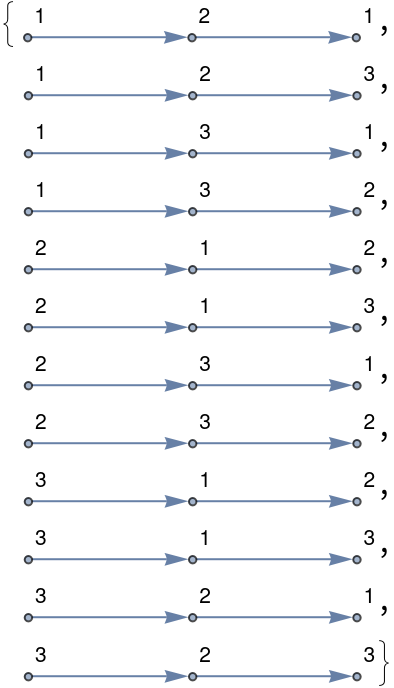FindProperColorings works on multigraphs:

 In:=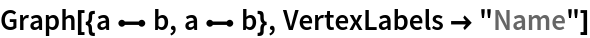Out=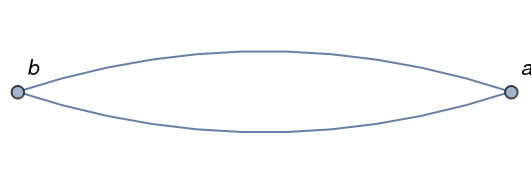In:=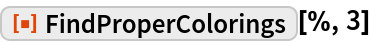Out=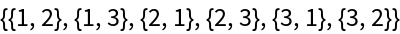In:=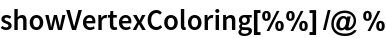Out=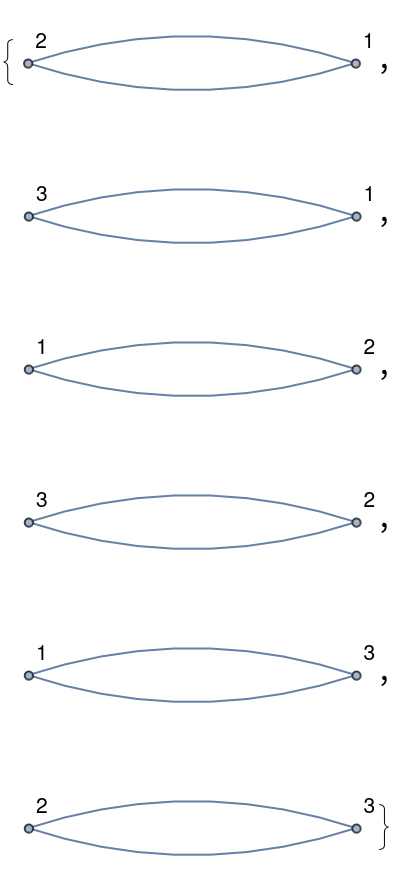### Applications

Define a graph:

 In:=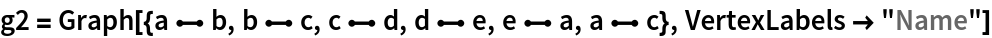Out=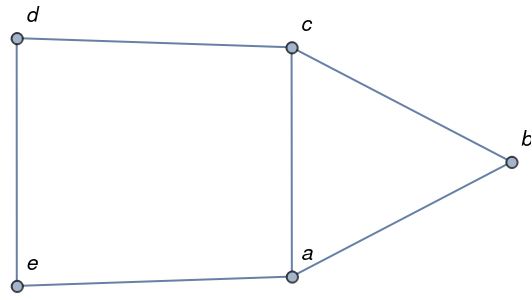A proper k-edge-coloring of a graph g is a function assigning each edge of g one of k colors such that incident edges receive distinct colors:

 In:=Find the proper 3-edge-colorings of the graph:

 In:=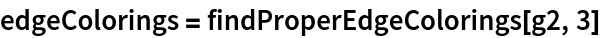Out=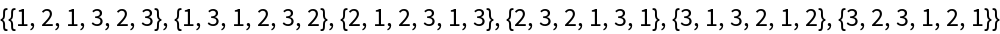Display the proper 3-edge-colorings:

 In:=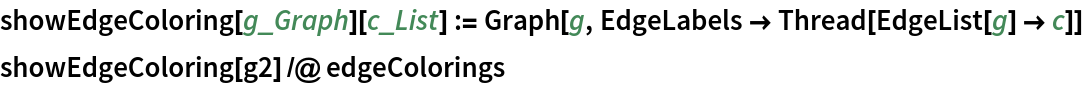Out=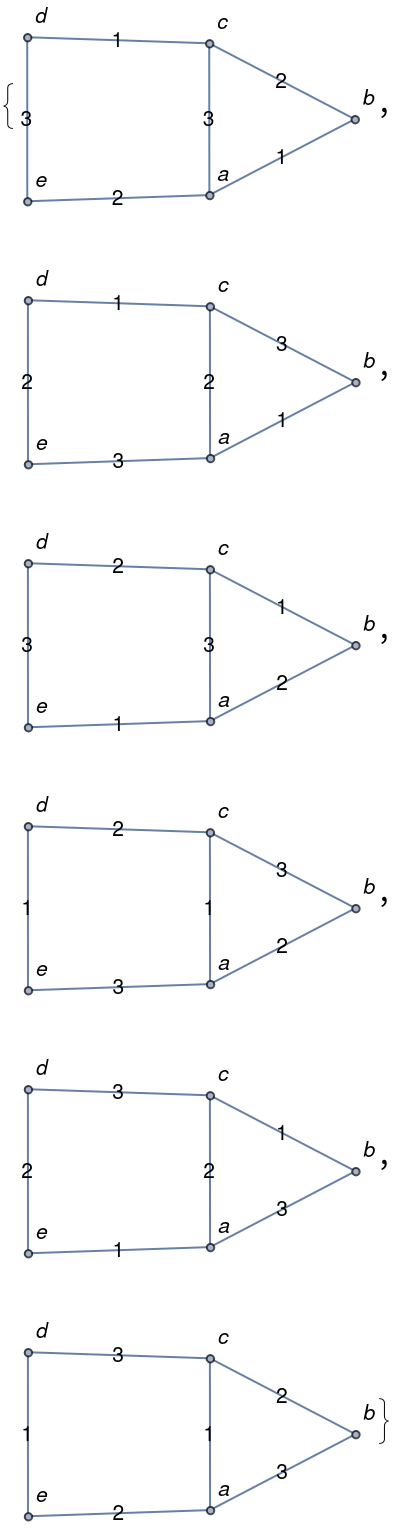### Properties and Relations

Define a graph:

 In:=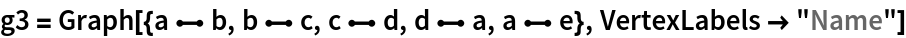Out=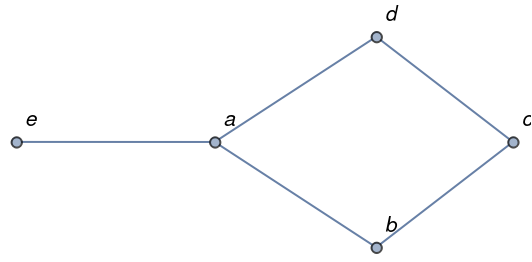The chromatic polynomial f of a graph g is a function such that f(k) counts the proper k-colorings of g:

 In:=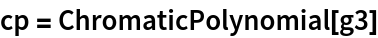Out=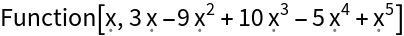Use the chromatic polynomial to count the proper k-colorings for 1≤k≤5:

 In:=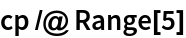Out=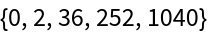Confirm that this matches the number of proper colorings returned by FindProperColorings:

 In:=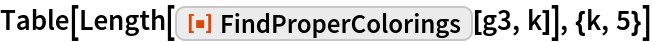Out=